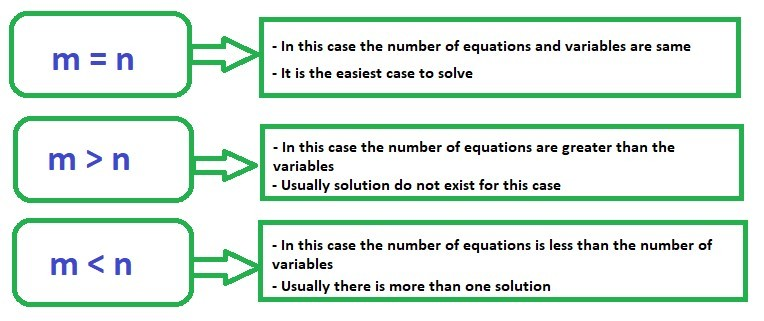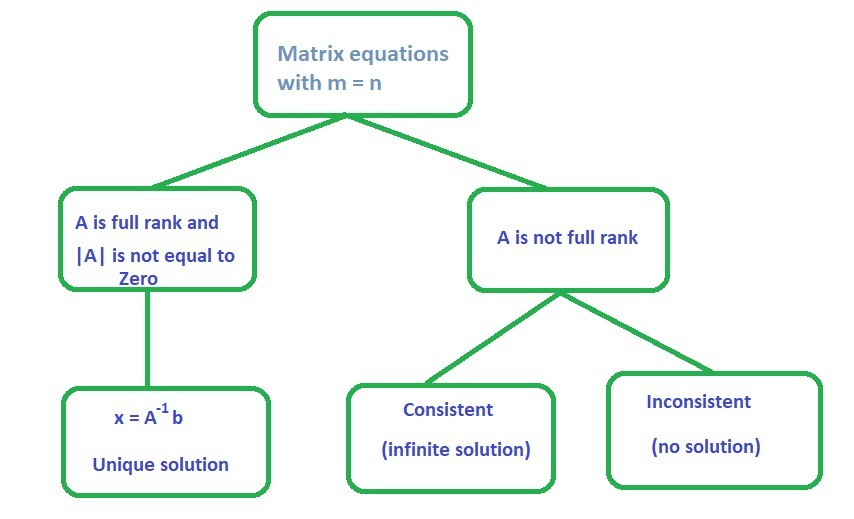# Data Science – Solving Linear Equations

Prerequisite: Introduction to Data Science : Skills Required

Linear Algebra is a very fundamental part of Data Science. When one talks about Data Science, data representation becomes an important aspect of Data Science. Data is represented usually in a matrix form. The second important thing in the perspective of Data Science is if this data contains several variables of interest, then one is interested to know how many of these are very important. And if there are relationships between these variables, then how can one uncover these relationships. Linear algebraic tools allow us to understand these data. So, a Data Science enthusiast needs to have a good understanding of this concept before going to understand complex machine learning algorithms.

Matrices and Linear Algebra
There are many ways to represent the data, matrices provide you with a convenient way to organize these data.

• Matrices can be used to represent samples with multiple attributes in a compact form
• Matrices can also be used to represent linear equations in a compact and simple fashion
• Linear algebra provides tools to understand and manipulate matrices to derive useful knowledge from data

Identification of Linear Relationships Among Attributes
We identify the linear relationship between attributes using the concept of null space and nullity. Before proceeding further, go through Null Space and Nullity of a Matrix.

Preliminaries

Generalized linear equations are represented as below:m and n are the number of equations and variables respectively
b is the general RHS commonly used


In general there are three cases one need to understand:We will consider these three cases independently.

Full row rank and full column rank
For a matrix A (m x n)

Full Row Rank Full Column Rank
When all the rows of the matrix are linearly independent When all the columns of the matrix are linearly independent
Data sampling does not present a linear relationship – samples are independent Attributes are linearly independent

Note: In general whatever be the size of the matrix it is established that row rank is always equal to the column rank. It means for any size of the matrix if we have certain number of independent rows, we will have those many numbers of independent column.
In general case if we have a matrix m x n and m is smaller than n then the maximum rank of the matrix can only be m. So, maximum rank is always the less of the two numbers m and n.

Case 1: m = nExample 1.1:

Consider the given matrix equation:
(1)|A| is not equal to zero
rank(A) = 2 = no. of columns

This implies that A is full rankTherefore, the solution for the given example isProgram to find rank and inverse of a matrix and solve the matrix equation in Python:

 # First, import  # matrix_rank from numpy.linalg  from numpy.linalg import matrix_rank, inv, solve     # A 2 x 2 matrix  A = [[1, 3],         [2, 4]]  b = [7, 10]      # Rank of matrix A  print("Rank of the matrix is:", matrix_rank(A))      # Inverse of matrix A  print("\nInverse of A:\n", inv(A))      # Matrix equation solution  print("Solution of linear equations:", solve(A, b))

Output:

Rank of the matrix is: 2

Inverse of A:
[[-2.   1.5]
[ 1.  -0.5]]

Solution of linear equation: [ 1.  2.]


You can refer Numpy | Linear Algebra article for various operations on matrix and to solve linear equations in Python.
Example 1.2:

Consider the given matrix equation:
(2)|A| is not equal to zero
rank(A) = 1
nullity = 1
Checking consistencyRow (2) = 2 Row (1)

The equations are consistent with only one linearly independent equation
The solution set for (,) is infinite because we have only
one linearly independent equation and two variables


Explanation: In the above example we have only one linearly independent equation i.e.. So, if we take, then we have; if we take, then we have. In the similar fashion we can have many solutions to this equation. We can take any value of( we have infinite choices for) and corespondingly for each value ofwe will get one. Hence, we can say that this equation has infinite solutions.

Example 1.3:

Consider the given matrix equation:
(3)|A| is not equal to zero
rank(A) = 1
nullity = 1
Checking consistency2 Row (1) =Therefore, the equations are inconsistent
We cannot find the solution to ()


Case 2: m > n

• In this case, the number of variables or the attributes is less than the number of equations.
• Here, not all the equations can be satisfied.
• So, it is sometimes termed as the case of no solution.
• But, we can try to identify an appropriate solution by viewing this case from optimization perspective.

An optimization perspective

- Rather than finding a solution to, we can find ansuch that ()
is minimized
- Here,is a vector
- There will be as many error terms as the number of equations
- Denote= e (m x 1); there are m errors, i = 1:m
- We can minimize all the errors collectively by minimizing- This is the same as minimizingSo, the optimization problem becomes==Here, we can notice that the optimization problem is a function of x. When we solve this optimization problem, it will give us the solution for x. We can obtain the solution to this optimization problem by differentiatingwith respect to x and setting the differential to zero.– Now, differentiating f(x) and setting the differential to zero results in– Assuming that all the columns are linearly independentNote: While this solution x might not satisfy all the equation but it will ensure that the errors in the equations are collectively minimized.

Example 2.1:

Consider the given matrix equation:
(4)m = 3, n = 2
Using the optimization conceptTherefore, the solution for the given linear equation isSubstituting in the equation showsExample 2.2:

Consider the given matrix equation:
(5)m = 3, n = 2
Using the optimization conceptTherefore, the solution for the given linear equation isSubstituting in the equation showsSo, the important poin to notice in the case 2 is that if we have more equations than variables then we can always use the least square solution which is. There is one thing to keep in mind is thatexists if the columns of A are linearly independent.

Case 3: m < n

• This case deals with more number of attributes or variables than equations
• Here, we can obtain multiple solutions for the attributes
• This is an infinite solution case
• We will see how we can choose one solution from the set of infinite possible solution
• In this case also we have an optimization perspective.Know what is Lagrange function here.
– Given below is the optimization problem

min()
such that,– We can define a Lagrangian function– Differentiate the Lagrangian with respect to x, and set it to zero, then we will get,Pre – multiplying by AFrom above we can obtainassuming that all the rows are linearly independentExample 3.1:

Consider the given matrix equation:
(6)m = 2, n = 3
Using the optimization concept,The solution for given sample is () = (-0.2, -0.4, 1)
You can easily verify thatGeneralization

• The above-described cases cover all the possible scenarios that one may encounter while solving linear equations.
• The concept we use to generalize the solutions for all the above cases is called Moore – Penrose Pseudoinverse of a matrix.
• Singular Value Decomposition can be used to calculate the psuedoinverse or the generalized inverse ().
• Attention geek! Strengthen your foundations with the Python Programming Foundation Course and learn the basics.

To begin with, your interview preparations Enhance your Data Structures concepts with the Python DS Course.

My Personal Notes arrow_drop_upPossess good Mathematical and Statistical Foundation Data Science Enthusiast Addicted to Python

If you like GeeksforGeeks and would like to contribute, you can also write an article using contribute.geeksforgeeks.org or mail your article to contribute@geeksforgeeks.org. See your article appearing on the GeeksforGeeks main page and help other Geeks.

Please Improve this article if you find anything incorrect by clicking on the "Improve Article" button below.